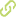# QFCS: A fuzzy LCS in continuous multi-step environments with continuous vector actions Chapter in Scopus•
• Overview
•
• Identity
•
•
• View All
•

### Abstract

• This paper introduces the QFCS, a new approach to fuzzy learning classifier systems. QFCS can solve the multistep reinforcement learning problem in continuous environments and with a set of continuous vector actions. Rules in the QFCS are small fuzzy systems. QFCS uses a Q-learning algorithm to learn the mapping between inputs and outputs. This paper presents results that show that QFCS can evolve rules to represent only those parts of the input and action space where the expected values are important for making decisions. Results for the QFCS are compared with those obtained by Q-learning with a high discretization to show that the new approach converges in a way similar to how Q-learning does for one-dimension problems with an optimal solution, and for two dimensions QFCS learns suboptimal solutions while it is difficult for Q-learning to converge due to that high discretization. © 2008 Springer-Verlag Berlin Heidelberg.

### Publication date

• November 26, 2008